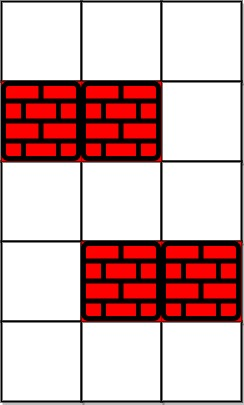# 1293. Shortest Path in a Grid with Obstacles Elimination

You are given an `m x n` integer matrix `grid` where each cell is either `0` (empty) or `1` (obstacle). You can move up, down, left, or right from and to an empty cell in one step.

Return  the minimum number of steps to walk from the upper left corner `(0, 0)`  to the lower right corner  `(m - 1, n - 1)`  given that you can eliminate at most  `k`  obstacles. If it is not possible to find such walk return `-1`.

Example 1:``````Input: grid = [[0,0,0],[1,1,0],[0,0,0],[0,1,1],[0,0,0]], k = 1
Output: 6
Explanation:
The shortest path without eliminating any obstacle is 10.
The shortest path with one obstacle elimination at position (3,2) is 6. Such path is (0,0) -> (0,1) -> (0,2) -> (1,2) -> (2,2) -> (3,2) -> (4,2).
``````

Example 2:``````Input: grid = [[0,1,1],[1,1,1],[1,0,0]], k = 1
Output: -1
Explanation: We need to eliminate at least two obstacles to find such a walk.
``````

Constraints:

• `m == grid.length`
• `n == grid[i].length`
• `1 <= m, n <= 40`
• `1 <= k <= m * n`
• `grid[i][j]` is either `0` or `1`.
• `grid == grid[m - 1][n - 1] == 0`

``````class Solution {
public:
int shortestPath(vector<vector<int>>& grid, int k) {
int res = 0, m = grid.size(), n = grid.size();
vector<vector<int>> dirs{{-1, 0}, {0, 1}, {1, 0}, {0, -1}};
vector<vector<int>> visited(m, vector<int>(n, -1)); // The number of obstacles that we can still remove after walking through that cell
visited = k;
queue<vector<int>> q;
q.push({0, 0, k});
while (!q.empty()) {
for (int i = q.size(); i > 0; --i) {
auto t = q.front(); q.pop();
if (t == m - 1 && t == n - 1) return res;
for (auto dir : dirs) {
int x = t + dir, y = t + dir;
if (x < 0 || x >= m || y < 0 || y >= n) continue;
int newK = t - grid[x][y];
if (newK < 0 || newK <= visited[x][y]) continue;
visited[x][y] = newK;
q.push({x, y, newK});
}
}
++res;
}
return -1;
}
};
``````

``````class Solution {
public:
int shortestPath(vector<vector<int>>& grid, int k) {
int res = 0, m = grid.size(), n = grid.size();
vector<vector<int>> dirs{{-1, 0}, {0, 1}, {1, 0}, {0, -1}};
vector<vector<int>> visited(m, vector<int>(n, INT_MAX)); // Record the minimum obstacles removed to get to that position
visited = 0;
queue<vector<int>> q;
q.push({0, 0, 0});
while (!q.empty()) {
for (int i = q.size(); i > 0; --i) {
auto t = q.front(); q.pop();
if (t == m - 1 && t == n - 1) return res;
for (auto dir : dirs) {
int x = t + dir, y = t + dir;
if (x < 0 || x >= m || y < 0 || y >= n) continue;
int newK = t + grid[x][y];
if (newK > k || visited[x][y] <= newK) continue;
visited[x][y] = newK;
q.push({x, y, newK});
}
}
++res;
}
return -1;
}
};
``````

Github 同步地址:

https://github.com/grandyang/leetcode/issues/1293

Shortest Path to Get Food

https://leetcode.com/problems/shortest-path-in-a-grid-with-obstacles-elimination/

https://leetcode.com/problems/shortest-path-in-a-grid-with-obstacles-elimination/discuss/712992/C%2B%2B-or-BFS

https://leetcode.com/problems/shortest-path-in-a-grid-with-obstacles-elimination/discuss/1188835/Java-Clean-O(MNK)-Time-BFS-Solution-oror-comparing-with-Dijkstra's

LeetCode All in One 题目讲解汇总(持续更新中…)

（欢迎加入博主的知识星球，博主将及时答疑解惑，并分享刷题经验与总结，快快加入吧～）|

Venmo 打赏—|—

 微信打赏Venmo 打赏（欢迎加入博主的知识星球，博主将及时答疑解惑，并分享刷题经验与总结，试运营期间前五十位可享受半价优惠～）×

Help us with donation